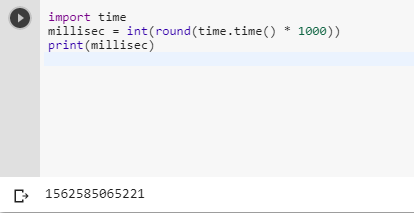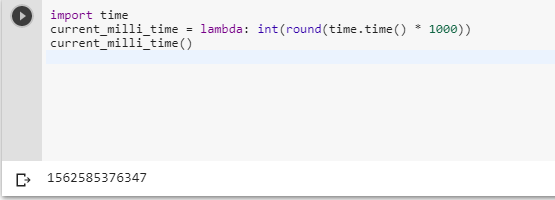3 views
in Python

How can I get the current time in milliseconds in Python?

by (106k points)

There are multiple ways of doing this problem some of the important methods I am discussing here:-

The first way we can solve this problem by using the time module and round() function:-

import time

millisec = int(round(time.time() * 1000))

print(millisec)Another method we have is to use the lambda function to solve this problem module:-

import time

current_milli_time = lambda: int(round(time.time() * 1000))

current_milli_time()